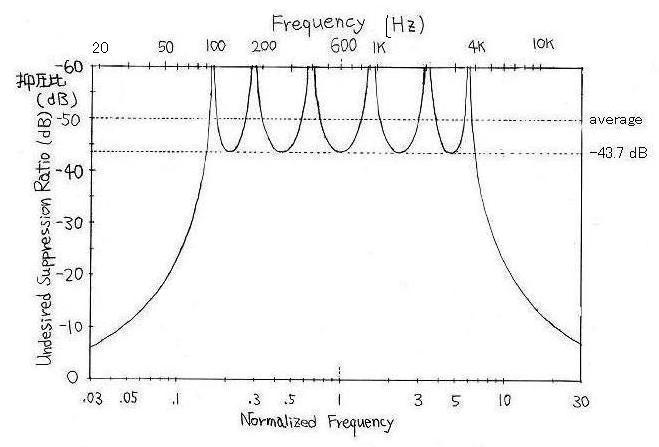# An Optimal Design of PSN for SSB Signals Generation and Reception I

Shigeo Yamase, JA7UHV

Summary:
One of the sixth order PSN (Phase Shift Network)'s has been optimized.

It has the following characteristics: the 6th order, 90 plus minus 0.750 degrees, and fh/fl = 44.17766746. The fh/fl is a ratio of the highest to the lowest in frequency where phase differece of the psn is maintained within 90 plus minus 0.750 degrees. The undesired sideband suppression ratio is -43.7 dB at each of the frequencies with the maximum phase deviation of 0.750 degrees from 90 degrees between fh and fl.

The average suppression of the undesired sideband over the bandwidth of modulating frequencies between fh and fl is 6.4 dB better than the suppression at each of the above frequencies.

Table 1

 Nr Phase Deviation from 90 degrees Normalized Frequency Example 1. Frequency in Hz 1 -0.750 fl = 0.1504522230 Fl = fl*F0 = 90.27 2 0.000 0.1646530906 98.79 3 +0.750 0.2099292534 126.0 4 0.000 0.2947908841 176.9 5 -0.750 0.4351162990 261.1 6 0.000 0.6568709219 394.1 7 +0.750 1.000000000 F0 = 600.0 8 0.000 1.522369109 913.4 9 -0.750 2.298236132 1379 10 0.000 3.392235153 2035 11 +0.750 4.763509535 2858 12 0.000 6.073375217 3644 13 -0.750 fh = 6.646628278 Fh = fh*F0 = 3988
Note: An asterisk * is a multiplier operator, like 12.3*2.0 = 24.6.Figure 1. Differential phase-shift characteristics of the network
The graph has a twofold symmetry, of which the axis is
on a normalized frequency of 1. So the left half of the graph
is the same as the right half with respect to the axis, as in a mirror.Figure 1-2. Undesired Sideband Suppression Ratio Characteristic of the network
The graph has a twofold symmetry.

Next